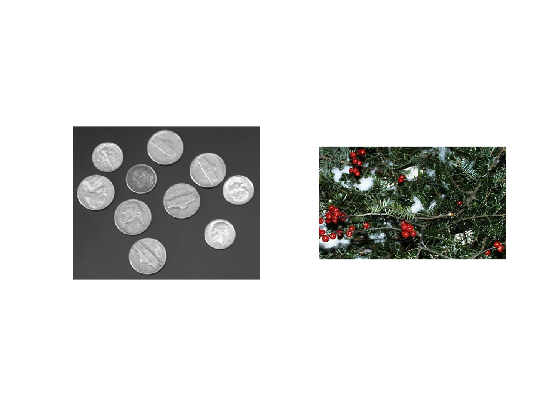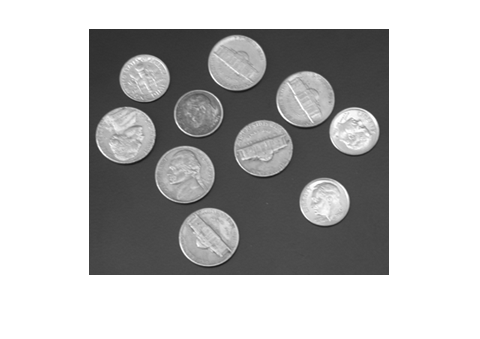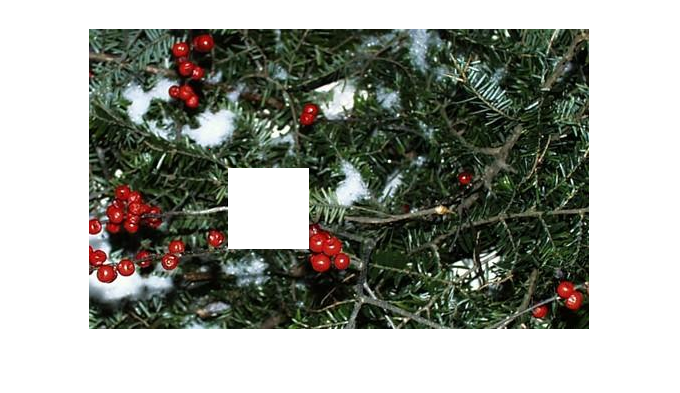# Image as matrix data in Matlab

Matlab allow us to load digital images and to manipulate the numbers associated with each pixel (color images RGB or gray images).
Usually color data are uint8 variables, therefore it is convenient to convert these numbers to double if products or divisions are involved in the data manipulation.

## Load and show an image

The Matlab functions imread and imshow allow us load images to Matlab
imRGB = imread('greens.jpg'); % a color image
imshow(imRGB)figure() %to show in another window
imGray = imread('coins.png'); % a gray image
imshow(imGray)%make a copy for later use
imRGBOrig= imRGB;
imGrayOrig= imGray;

## Manipulating an image

If you have already load an image you can acces the pixel values. To know its size you have to take into account 3 dimensions: witdh x height x depth. Being depth 1 or 3 according to color or gray images.
[m,n,p]=size(imRGB);
[mm,nn,pp]=size(imGray);
p121RGB = imRGB(121,121,:);
fprintf('colors RGB = %d %d %d \n', p121RGB); %print in the command window
colors RGB = 23 46 28
p121Gray = imGray(121,121,:);
fprintf('color Gray = %d \n', p121Gray); %print in the command window
color Gray = 68
% modify a pixel value (to red)
imRGB(121,121,1) = 255; %assign max red color channel
imRGB(121,121,2) = 0; %assign min green color channel
imRGB(121,121,3) = 0; %assign min blue color channel
% if you have only a channel (to white)
imGray(121,121,1) = 255; %assign max red color channel
% We can also modify a set of pixels like a matrix
%
imRGB(140:220,140:220,:)=255; %white square
imshow(imRGB)## Manipulate according color level

We will change the red pixels (until a certain level) to black. All three channels are explored before changing the color.
imRGB = imRGBOrig; % recover original values
for i=1:m
for j=1:n
if ( (imRGBOrig(i,j,1)> 100) && (imRGBOrig(i,j,2) < 80) && (imRGBOrig(i,j,3) < 80))
imRGB(i,j,:)=[0,0,0];
end
end
end
figure()
imshow(imRGB);